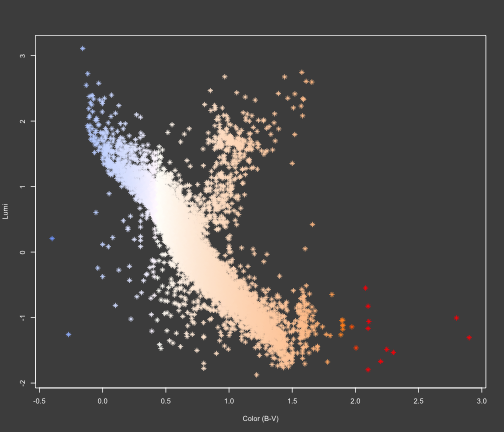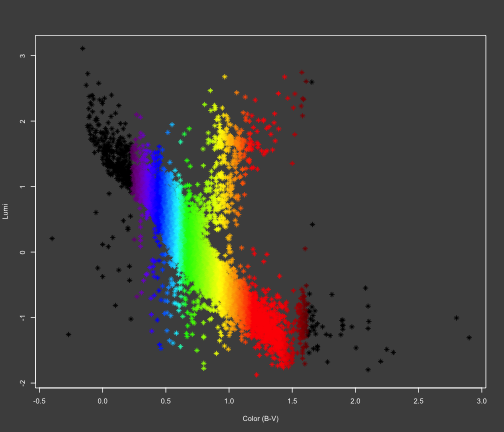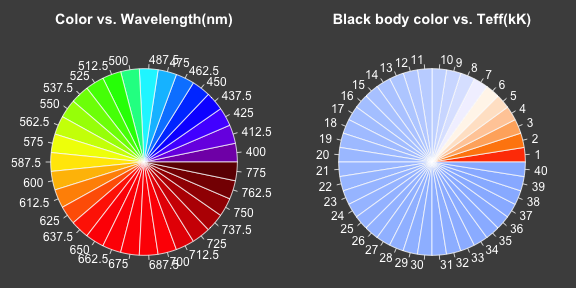# Reproducing the Hertzsprung-Russell diagram with R

As a plotting exercise in R, we try to reproduce the Hertzsprung-Russell diagram. We first download the data from the Hipparcos catalogue using the VizieR interface. Since the data is quite large, we can bzip2 the output tsv file.

Now let's run our code:

``````inputfile <- "~/work/teaching/phys443/R/classwork/vizier.tsv.bz2"
source("~/work/teaching/phys443/R/classwork/hrdiag.R")
data <- hrdiag(inputfile, 15, 30)  # for stars with parallax between 15 and 30
``````
``````##  "Nstars = " "8814"
``````The plot has ```proper" black-body coloring for stars. If we want```fake" coloring as is sometimes shown on HR plots on the web, ie. only the color of the wavelength at which the star emits most (according to Wien's displacement law), we can do the following:

``````plotHRdiag(data, TRUE)
``````The colors were obtained through two functions, here are the wheels for them:

``````par(mar = c(0, 0, 2.5, 0), cex = 0.6, bg = gray.colors(1), col.axis = "white",
col.lab = "white", fg = "white", col.main = "white", mfrow = c(1, 2))
showVisibleColor()
showStarColor()
``````### References

Most of the code for this example is based on what was found on the web. Please see the source for the links.